https://mooc.study.163.com/smartSpec/detail/1001319001.htm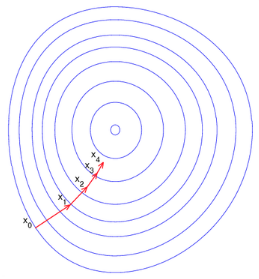$w=w-\alpha* dw$

## Momentum算法

Momentum算法又叫做冲量算法，其迭代更新公式如下：

$\begin{cases} v=\beta v+(1-\beta)dw \\ w=w-\alpha v \end{cases}$

### 指数加权平均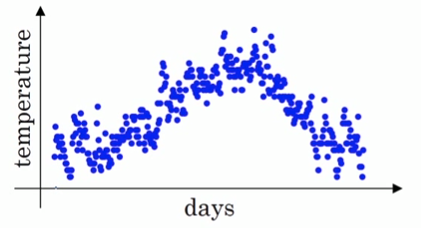$\begin{cases} v_0=0 \\ v_k=\beta v_{k-1}+(1-\beta)\theta_k, \quad k=1,2,...,365 \end{cases}$

v就是指数加权平均值，也就是平滑后的气温。$\beta$的典型值是0.9，平滑后的曲线如下图所示：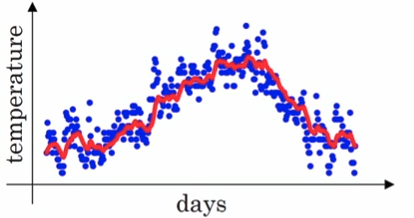$\begin{split} v_k&=\beta v_{k-1}+(1-\beta)\theta_k \\ &=\beta^kv_0+\beta^{k-1}(1-\beta)\theta_1+\beta^{k-2}(1-\beta)\theta_2+\dots+\beta(1-\beta)\theta_{k-1}+(1-\beta)\theta_k \\ &=\beta^{k-1}(1-\beta)\theta_1+\beta^{k-2}(1-\beta)\theta_2+\dots+\beta(1-\beta)\theta_{k-1}+(1-\beta)\theta_k \end{split}$

$v_k=\frac{\beta v_{k-1}+(1-\beta)\theta_k}{1-\beta^k}$

$1-\beta^k$是所有权重的和，这相当于对权重做了一个归一化处理。下面的图中，紫色的线就是没有做修正的结果，修正之后就是绿色曲线。二者在前面几个数据点之间相差较大，后面则基本重合了。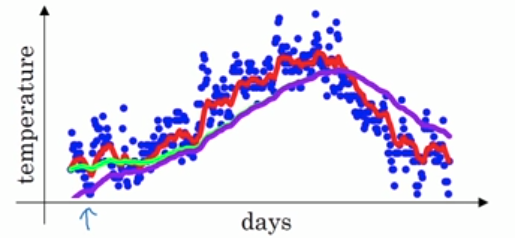### 回看Momentum算法

$\begin{cases} v=\beta v+(1-\beta)dw \\ w=w-\alpha v \end{cases}$

$dw$是我们计算出来的原始梯度，$v$则是用指数加权平均计算出来的梯度。这相当于对原始梯度做了一个平滑，然后再用来做梯度下降。实验表明，相比于标准梯度下降算法，Momentum算法具有更快的收敛速度。为什么呢？看下面的图，蓝线是标准梯度下降法，可以看到收敛过程中产生了一些震荡。这些震荡在纵轴方向上是均匀的，几乎可以相互抵消，也就是说如果直接沿着横轴方向迭代，收敛速度可以加快。Momentum通过对原始梯度做了一个平滑，正好将纵轴方向的梯度抹平了（红线部分），使得参数更新方向更多地沿着横轴进行，因此速度更快。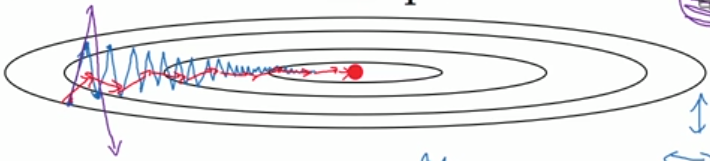## RMSprop算法

$\begin{cases} s_1=\beta_1 s_1+(1-\beta_1)dw_1^2 \\ s_2=\beta_2 s_2+(1-\beta_2)dw_2^2 \end{cases}$

$\begin{cases} w_1=w_1-\alpha \frac{dw_1}{\sqrt{s_1+\epsilon}} \\ w_2=w_2-\alpha \frac{dw_2}{\sqrt{s_2+\epsilon}} \end{cases}$

$\begin{cases} s=\beta s+(1-\beta)dw^2 \\ w=w-\alpha\frac{dw}{\sqrt{s+\epsilon}} \end{cases}$

$\beta$的典型值是0.999。公式中还有一个$\epsilon$，这是一个很小的数，典型值是$10^{-8}$

$\begin{cases} v=\beta_1 v+(1-\beta_1)dw \\ s=\beta_2 s+(1-\beta_2)dw^2 \\ w=w-\alpha\frac{v}{\sqrt{s+\epsilon}} \end{cases}$